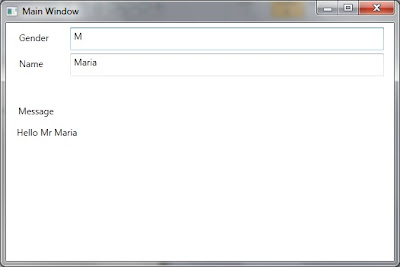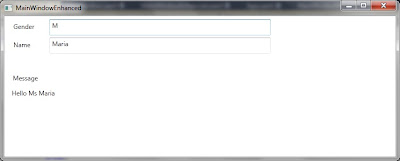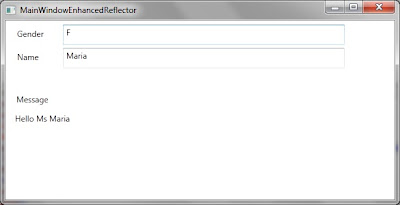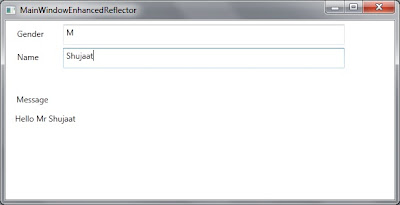## Friday, February 4, 2011

### WPF - Binding Converter Parameter [Including Discussion about Binding Reflector]

In this post we will be discussing how we can bind ConverterParameter used in WPF Binding. As we know that ConverterParameter is not a DependencyProperty, we can not bind it directly. We will be looking at the possible workarounds to achieve the similar results. As always, we will start with a simple window and then add necessary ingredients for this discussion. Let us create a sample Window with two text boxes for entering Gender and Name of a Student.
`<Window x:Class="WpfApplication_ConverterParameterBinding.MainWindow"        xmlns="http://schemas.microsoft.com/winfx/2006/xaml/presentation"        xmlns:x="http://schemas.microsoft.com/winfx/2006/xaml"        xmlns:local="clr-namespace:WpfApplication_ConverterParameterBinding"        Title="Main Window" Height="350" Width="525">    <Window.DataContext>        <local:MainWindowViewModel />           </Window.DataContext>    <Grid>        <TextBox Height="30" HorizontalAlignment="Left" Margin="84,6,0,0" Name="textBoxGender"                  VerticalAlignment="Top" Width="412" >            <TextBox.Text>                <Binding Path="Gender" UpdateSourceTrigger="PropertyChanged" Mode="TwoWay" />             </TextBox.Text>        </TextBox>        <Label Content="Gender" Height="28" HorizontalAlignment="Left" Margin="12,6,0,0"               Name="labelGender" VerticalAlignment="Top" Width="58" />        <TextBox Height="30" HorizontalAlignment="Left" Margin="84,40,0,0" Name="textBoxStudentName"                 VerticalAlignment="Top" Width="412" >            <TextBox.Text>                <Binding Path="StudentName" UpdateSourceTrigger="PropertyChanged" Mode="TwoWay" />            </TextBox.Text>        </TextBox>        <Label Content="Name" Height="28" HorizontalAlignment="Left" Margin="12,40,0,0"                Name="labelStudentName" VerticalAlignment="Top" Width="58" />    </Grid></Window>`

As you can see that we have used an instance of MainWindowViewModel as DataContext of the Window. MainWindowViewModel is presented as below:
`namespace WpfApplication_ConverterParameterBinding{    using System.Windows;    class MainWindowViewModel : DependencyObject    {        public static DependencyProperty GenderProperty =          DependencyProperty.Register("Gender", typeof(string),          typeof(MainWindowViewModel), new FrameworkPropertyMetadata() { AffectsRender = true });        public string Gender        {            get { return (string)GetValue(GenderProperty); }            set { SetValue(GenderProperty, value); }        }        public static DependencyProperty StudentNameProperty =          DependencyProperty.Register("StudentName", typeof(string),          typeof(MainWindowViewModel), new FrameworkPropertyMetadata() { AffectsRender = true });        public string StudentName        {            get { return (string)GetValue(StudentNameProperty); }            set { SetValue(StudentNameProperty, value); }        }    }}`

When we run this application, it appears as follows:Now we want to display a Welcome message to the Student based on the information entered. If it is a Male student (Gender = M), the message should be read as "Welcome Mr. NAME OF STUDENT". If it is a female student (Gender = F), it should read as "Welcome Ms. NAME OF STUDENT".In order to update the Window, let us add a TextBlock at the bottom as follows:
`<Window x:Class="WpfApplication_ConverterParameterBinding.MainWindow"        xmlns="http://schemas.microsoft.com/winfx/2006/xaml/presentation"        xmlns:x="http://schemas.microsoft.com/winfx/2006/xaml"        xmlns:local="clr-namespace:WpfApplication_ConverterParameterBinding"        Title="Main Window" Height="350" Width="525">    <Window.DataContext>        <local:MainWindowViewModel />           </Window.DataContext>    <Grid>        <TextBox Height="30" HorizontalAlignment="Left" Margin="84,6,0,0" Name="textBoxGender"                  VerticalAlignment="Top" Width="412" >            <TextBox.Text>                <Binding Path="Gender" UpdateSourceTrigger="PropertyChanged" Mode="TwoWay" />             </TextBox.Text>        </TextBox>        <Label Content="Gender" Height="28" HorizontalAlignment="Left" Margin="12,6,0,0"               Name="labelGender" VerticalAlignment="Top" Width="58" />        <TextBox Height="30" HorizontalAlignment="Left" Margin="84,40,0,0" Name="textBoxStudentName"                 VerticalAlignment="Top" Width="412" >            <TextBox.Text>                <Binding Path="StudentName" UpdateSourceTrigger="PropertyChanged" Mode="TwoWay" />            </TextBox.Text>        </TextBox>        <Label Content="Name" Height="28" HorizontalAlignment="Left" Margin="12,40,0,0"                Name="labelStudentName" VerticalAlignment="Top" Width="58" />        <TextBlock Height="164" HorizontalAlignment="Left" Margin="14,135,0,0" Name="textBlockMessage"                 Text="Hello Mr. Muhammad"  VerticalAlignment="Top" Width="479" />        <Label Content="Message" Height="29" HorizontalAlignment="Left" Margin="11,102,0,0"               Name="label1" VerticalAlignment="Top" Width="129" />    </Grid></Window>`

The text is hardcode "Hello Mr. Muhammad. We want it to be based on the Data entered. First let us update the Binding that it just shows "Hello NAME OF STUDENT" message in the notification box.
`<TextBlock Height="164" HorizontalAlignment="Left" Margin="14,135,0,0" Name="textBlockMessage"        VerticalAlignment="Top" Width="479" >    <TextBlock.Text>        <Binding Path="StudentName" StringFormat="Hello {0}" />    </TextBlock.Text></TextBlock>`

Now when we run the application, it shows a welcome message to the student.Since we want to show the salutation (Mr. / Ms.) with name so we need to somehow use Gender. if it is M, we should show "Welcome Mr. NAME OF STUDENT". On the other hand if it is F, we should show "Hello Ms. NAME OF STUDENT".
Let us define an IValueConverter for this purpose:
`namespace WpfApplication_ConverterParameterBinding{    using System;    using System.Windows.Data;    class MessageConverter : IValueConverter    {        public object Convert(object value, Type targetType, object parameter, System.Globalization.CultureInfo culture)        {            string salutation = (parameter == null || parameter == "M") ? "Mr." : "Ms";            return string.Format("Hello {0} {1}", salutation, value);        }        public object ConvertBack(object value, Type targetType, object parameter, System.Globalization.CultureInfo culture)        {            throw new NotImplementedException();        }    }}`

Basically we are expecting that, somehow, we would be able to pass Gender as parameter of Converter. Let us instantiate the Converter in the Resources section of the window.
`<Window.Resources>    <local:MessageConverter x:Key="messageConverter" /></Window.Resources>`

This is used for Binding the converter as follows:
`<TextBlock.Text>    <Binding Path="StudentName" Converter="{StaticResource messageConverter}" ConverterParameter="{Binding Gender}" /></TextBlock.Text>`

This seems to pass Gender for ConverterParameter for Binding. But as we run the applcation, it results in an exception.This is because of the limitation as mentioned in the beginning of this post. Basically ConverterParameter is not a dependency property so we can not bind it. Now let us discuss the alternative solutions.

Using MultiBinding:
One way to resolve the issue of Binding ConverterParameter is by using MultiBinding. Instead of Binding ConverterParameter, just have a separate binding for Target property using MultiBinding. We would be needing MultiValueConverter for this purpose. We are expecting that the following converter would be used for MultiBinding (implements IMultiValueConverter) of Gender and Name property. If the values of none of these are available then return empty string. If any of these is available then greet the person [Hello Mr. NAME_OF_PERSON for Gender = M and Hello Ms. NAME_OF_PERSON for Gender = F]
`namespace WpfApplication_ConverterParameterBinding{    using System;    using System.Windows.Data;    class MessageMultiConverter : IMultiValueConverter    {        public object Convert(object[] values, Type targetType, object parameter, System.Globalization.CultureInfo culture)        {            string name = (values == null) ? string.Empty : values.ToString();            string gender = (values == null) ? string.Empty : values.ToString();                                    string salutation;            switch(gender)            {                case "M":                    salutation = "Mr";                    break;                case "F":                    salutation = "Ms";                    break;                default:                    salutation = string.Empty;                    break;            }            string message = string.Empty;            if (!(name == string.Empty && gender == string.Empty))            {                 message = string.Format("Hello {0} {1}", salutation, name);            }                                    return message;        }        public object[] ConvertBack(object value, Type[] targetTypes, object parameter, System.Globalization.CultureInfo culture)        {            throw new NotImplementedException();        }    }}`

Let's use this converter in a view. The only update is the usage of this new converter. We are instantiating it in the Resources section of Window. We are using the same converter with text block showing Message.
`<Window x:Class="WpfApplication_ConverterParameterBinding.MainWindowMultiBinding"        xmlns="http://schemas.microsoft.com/winfx/2006/xaml/presentation"        xmlns:x="http://schemas.microsoft.com/winfx/2006/xaml"        xmlns:local="clr-namespace:WpfApplication_ConverterParameterBinding"        Title="Main Window" Height="350" Width="525">    <Window.Resources>        <local:MessageMultiConverter x:Key="messageConverter" />    </Window.Resources>    <Window.DataContext>        <local:MainWindowViewModel />    </Window.DataContext>    <Grid>        <TextBox Height="30" HorizontalAlignment="Left" Margin="84,6,0,0" Name="textBoxGender"                  VerticalAlignment="Top" Width="412" >            <TextBox.Text>                <Binding Path="Gender" UpdateSourceTrigger="PropertyChanged" Mode="TwoWay" />            </TextBox.Text>        </TextBox>        <Label Content="Gender" Height="28" HorizontalAlignment="Left" Margin="12,6,0,0"               Name="labelGender" VerticalAlignment="Top" Width="58" />        <TextBox Height="30" HorizontalAlignment="Left" Margin="84,40,0,0" Name="textBoxStudentName"                 VerticalAlignment="Top" Width="412" >            <TextBox.Text>                <Binding Path="StudentName" UpdateSourceTrigger="PropertyChanged" Mode="TwoWay" />            </TextBox.Text>        </TextBox>        <Label Content="Name" Height="28" HorizontalAlignment="Left" Margin="12,40,0,0"                Name="labelStudentName" VerticalAlignment="Top" Width="58" />        <TextBlock Height="164" HorizontalAlignment="Left" Margin="14,135,0,0" Name="textBlockMessage"                VerticalAlignment="Top" Width="479" >            <TextBlock.Text>                <MultiBinding Converter="{StaticResource messageConverter}">                                        <Binding Path="Gender" />                    <Binding Path="StudentName" />                </MultiBinding>                            </TextBlock.Text>        </TextBlock>        <Label Content="Message" Height="29" HorizontalAlignment="Left" Margin="11,102,0,0"               Name="label1" VerticalAlignment="Top" Width="129" />    </Grid></Window>`

When we run the project, it appears as follows:As you can see that empty message is displayed. Let's enter F for Gender and Maria for name. It would update the message as follows:This might or might not be a requirement but let me explain what is going on. The re-evaluation of Converter is being done when either of Gender or Name of Student is updated. Since Gender is supposed to be used just as a ConverterParameter originally this re-evaluation might not be a desired behavior. It might be desired that converter is re-evaulated just when the actual intended bound property is updated. This update should just use the current value of the property specified as ConverterParameter. In order to prove this point just update the Gender to M. This would update the message as Hello Mr. Maria. This might not be desired.Introducing a Dependency Property in Converter and Using Binding Reflector:
ConverterParameter is a property of Binding. It is provided as a parameter to Convert and ConvertBack method of Converter whenever any update in Source or Target of Binding takes place.

Let us update define a new Converter. It is an IValueConverter like our first example. It just has an additional DependencyProperty. We name this as BindableConverterParameter.
`namespace WpfApplication_ConverterParameterBinding{    using System;    using System.Windows.Data;    using System.Windows;    class EnhancedMessageConverter : DependencyObject, IValueConverter    {        public static DependencyProperty BindableConverterParameterProperty =             DependencyProperty.Register("BindableConverterParameter", typeof(string),             typeof(EnhancedMessageConverter));        public string BindableConverterParameter        {            get { return (string)GetValue(BindableConverterParameterProperty); }            set { SetValue(BindableConverterParameterProperty, value); }        }        public object Convert(object value, Type targetType, object parameter, System.Globalization.CultureInfo culture)        {            string gender = BindableConverterParameter ?? string.Empty;            var name = value ?? string.Empty;            string salutation;            switch (gender)            {                case "M":                    salutation = "Mr";                    break;                case "F":                    salutation = "Ms";                    break;                default:                    salutation = string.Empty;                    break;            }            string message = string.Empty;            if (!(name == string.Empty && gender == string.Empty))            {                message = string.Format("Hello {0} {1}", salutation, name);            }            return message;                    }        public object ConvertBack(object value, Type targetType, object parameter, System.Globalization.CultureInfo culture)        {            throw new NotImplementedException();        }    }}`

Let's use this converter in the view.
`<Window x:Class="WpfApplication_ConverterParameterBinding.MainWindowEnhanced"        xmlns="http://schemas.microsoft.com/winfx/2006/xaml/presentation"        xmlns:x="http://schemas.microsoft.com/winfx/2006/xaml"        xmlns:local="clr-namespace:WpfApplication_ConverterParameterBinding"        Title="MainWindowEnhanced" Height="300" Width="300">    <Window.Resources>        <local:EnhancedMessageConverter x:Key="messageConverter"                                         BindableConverterParameter="{Binding Gender}" />    </Window.Resources>    <Window.DataContext>        <local:MainWindowViewModel />    </Window.DataContext>    <Grid>        <TextBox Height="30" HorizontalAlignment="Left" Margin="84,6,0,0" Name="textBoxGender"                  VerticalAlignment="Top" Width="412" >            <TextBox.Text>                <Binding Path="Gender" UpdateSourceTrigger="PropertyChanged" Mode="TwoWay" />            </TextBox.Text>        </TextBox>        <Label Content="Gender" Height="28" HorizontalAlignment="Left" Margin="12,6,0,0"               Name="labelGender" VerticalAlignment="Top" Width="58" />        <TextBox Height="30" HorizontalAlignment="Left" Margin="84,40,0,0" Name="textBoxStudentName"                 VerticalAlignment="Top" Width="412" >            <TextBox.Text>                <Binding Path="StudentName" UpdateSourceTrigger="PropertyChanged" Mode="TwoWay" />            </TextBox.Text>        </TextBox>        <Label Content="Name" Height="28" HorizontalAlignment="Left" Margin="12,40,0,0"                Name="labelStudentName" VerticalAlignment="Top" Width="58" />        <TextBlock Height="164" HorizontalAlignment="Left" Margin="14,135,0,0" Name="textBlockMessage"                VerticalAlignment="Top" Width="479" >           <TextBlock.Text>               <Binding Path="StudentName" Converter="{StaticResource messageConverter}" />           </TextBlock.Text>        </TextBlock>        <Label Content="Message" Height="29" HorizontalAlignment="Left" Margin="11,102,0,0"               Name="label1" VerticalAlignment="Top" Width="129" />    </Grid></Window>`

Just look at the instantiation of the Converter in Window.Resources section. BindableConverterParameter in initialized to be bound to Gender property of the object. From our knowledge of Data Binding in WPF, we know that if we don't provide any Binding Source then it would be considered as DataContext.Now keep changing the Gender and Name of Student in the view. No matter how many times we change the values entered, salutation title (Mr. / Ms.) never appears in the message text. As we see the definition of converter we know that this is only possible when BindableConverterParameter is null / Empty. Let's insert a break-point in the Convert method keep changing the data on the view.This is as per the expectation. Basically BindableConverterParameter is always null. This is basically due to perceived over-simplification of the problem. We are binding to the DataContext in the Resources section. It must be remembered that DataContext is a DependencyProperty inherited through the Visual Tree. Since Resources section is not in the Visual Tree, it would not have the DataContext inherited resulting in no Binding at all. Because of same reason Relative Source Binding would also not work if we attempt to do it like this:
`<Window.Resources>    <local:EnhancedMessageConverter x:Key="messageConverter"                                     BindableConverterParameter="{Binding RelativeSource={RelativeSource Self}, Path=DataContext.Gender}" /></Window.Resources>`

As we have discussed that Resources can not use DataContext property of the view. But we know that DataContext can utilize any view it desires. Let us try to Bind the BindableConverterParameter to Gender property of DataContext in such a way that Binding is defined on DataContext in reverse direction. This can be done as follows:
`<Window.Resources>    <local:EnhancedMessageConverter x:Key="messageConverter" /></Window.Resources><Window.DataContext>    <local:MainWindowViewModel>        <local:MainWindowViewModel.Gender>            <Binding Source="{StaticResource messageConverter}" Path="BindableConverterParameter" Mode="OneWayToSource" />        </local:MainWindowViewModel.Gender>    </local:MainWindowViewModel> </Window.DataContext>`

Now let us run the application. If we enter data in the text boxes, we realize that it results in update of the message to user. The greatest thing is that updates in Gender (used as ConverterParameter) is not causing re-evaluation of message. Message is re-evaluated only when the actual bound value to the message text block (StudentName) is updated. When we run the application and enter data [Gender=F and Name = Maria], the message is displayed as follows:Updating the Gender to M does not result in updating the display of message as follows:As we update the name of student, the updated value of BindableConverterParameter is considered and view is updated with new message as follows:Using Binding Reflector:
This is still not perfect solution because this is based on the assumption that view model is always instantiated by view. This is also possible that view model is instantiate separately and some other code assigns it to the DataContext property of view. Our logic would find limitation under this condition. In this case we are still instantiating the Converter in Resources section which is generally the norm.

The best solution could be to bind the Text property of Gender text box to BindableConverterParameter property of the Converter in OneWayToSource mode. But again, this is already bound to the Gender property of DataContext.

In WPF, a DependencyProperty might be the source for a number of Binding but it might be the Target of only one Binding. Although because of availability of various Binding modes, the difference between Source and Target of Binding in the real sense is really vague but Target is referred to the Property on which the binding is defined and Source is considered as the property which is actually being used for Binding to Target.

Based on the above discussion, this seems to be the end of world. But never lose hope! Let us invent a new tool. We name this tool as Binding Reflector. We will be using this to pass the updates in one dependency property to another Dependency Property. In our example we will be using it to Bind between Gender property of DataContext to BindableConverterParameter property of Converter instantiated in Resources section of Window. In order to use the DataContext, it must be an element in the Visual Tree of view. Let's define it as a FrameworkElement.
`namespace WpfApplication_ConverterParameterBinding{    using System.Windows;    using System.Windows.Data;    class BindingReflector : FrameworkElement    {        public static DependencyProperty SourceProperty =            DependencyProperty.Register("Source", typeof(object), typeof(BindingReflector),            new FrameworkPropertyMetadata()            {                DefaultUpdateSourceTrigger = UpdateSourceTrigger.PropertyChanged,                PropertyChangedCallback = OnSourceChanged            });        public object Source        {            get { return GetValue(SourceProperty); }            set { SetValue(SourceProperty, value); }        }        public static DependencyProperty TargetProperty =            DependencyProperty.Register("Target", typeof(object), typeof(BindingReflector),            new FrameworkPropertyMetadata() { DefaultUpdateSourceTrigger = UpdateSourceTrigger.PropertyChanged });        public object Target        {            get { return GetValue(TargetProperty); }            set { SetValue(TargetProperty, value); }        }        private static void OnSourceChanged(DependencyObject d, DependencyPropertyChangedEventArgs e)        {            var reflector = (BindingReflector)d;            if (reflector.Source != reflector.Target)            {                reflector.Target = reflector.Source;            }        }    }}`

As you can see that it is a FrameworkElement with just two Dependency properties i.e. Source and Target. Source is registered with a PropertyChangedCallBack. In the callback handler, we are just copying the value of Source Property to Target property. This Binding Reflector will be used in the view. With appropriate Binding, we will be obtaining the actual desired result. Let us define a new view as follows:
`<Window x:Class="WpfApplication_ConverterParameterBinding.MainWindowEnhancedReflector"        xmlns="http://schemas.microsoft.com/winfx/2006/xaml/presentation"        xmlns:x="http://schemas.microsoft.com/winfx/2006/xaml"        xmlns:local="clr-namespace:WpfApplication_ConverterParameterBinding"        Title="MainWindowEnhancedReflector" Height="300" Width="585">    <Window.Resources>        <local:EnhancedMessageConverter x:Key="messageConverter" />    </Window.Resources>    <Window.DataContext>        <local:MainWindowViewModelEnhanced />    </Window.DataContext>    <Grid>        <local:BindingReflector Target="{Binding  Mode=OneWayToSource, Source = {StaticResource messageConverter}, Path=BindableConverterParameter}"            Source="{Binding Path=Gender, Mode=OneWay}" />        <TextBox Height="30" HorizontalAlignment="Left" Margin="84,6,0,0" Name="textBoxGender"                  VerticalAlignment="Top" Width="412" >            <TextBox.Text>                <Binding Path="Gender" UpdateSourceTrigger="PropertyChanged" Mode="TwoWay" />            </TextBox.Text>        </TextBox>        <Label Content="Gender" Height="28" HorizontalAlignment="Left" Margin="12,6,0,0"               Name="labelGender" VerticalAlignment="Top" Width="58" />        <TextBox Height="30" HorizontalAlignment="Left" Margin="84,40,0,0" Name="textBoxStudentName"                 VerticalAlignment="Top" Width="412" >            <TextBox.Text>                <Binding Path="StudentName" UpdateSourceTrigger="PropertyChanged" Mode="TwoWay" />            </TextBox.Text>        </TextBox>        <Label Content="Name" Height="28" HorizontalAlignment="Left" Margin="12,40,0,0"                Name="labelStudentName" VerticalAlignment="Top" Width="58" />        <TextBlock Height="164" HorizontalAlignment="Left" Margin="14,135,0,0" Name="textBlockMessage"                VerticalAlignment="Top" Width="479" >           <TextBlock.Text>               <Binding Path="StudentName" Converter="{StaticResource messageConverter}" />           </TextBlock.Text>        </TextBlock>        <Label Content="Message" Height="29" HorizontalAlignment="Left" Margin="11,102,0,0"               Name="label1" VerticalAlignment="Top" Width="129" />    </Grid></Window>`

Just have a look at the first child of Grid. It is the same BindingReflector that we have created. You can see that we have used specific Binding modes for Source and Target Binding. For Source, it is One Way Binding so that it could receive value updates from Source. For Target property, it is set as OneWayToSource so that value changes as assigned in SourceChanged call back could be passed to the Target.

One important point is that we have bound Target property before Source property here. This is deliberate action. Basically as we know that using a FrameworkElement like this causes the default constructor of FramworkElement to be executed. It then assigns the specified properties to the values as specified. If we specify Source before Target here then the initial values of Source will be copied to the Target but they wouldn't be assigned to the Binding defined later. But if we first define binding for Target then as we would update the value of Target it would be available in BindableConverterParameter.

Now we run the application. The behavior is similar to our previous example. It is also not causing the Binding to re-evaluate when just BindableConverterParameter is updated. It is only updated when the actual Binding Source i.e. StudentName is updated. Let's run this to see this behavior:Now update Gender followed by Name and see the message updated as follows:Note:
In this post, we have introduced Binding Reflector. We can also use it to bind between dependency properties of two different Resources.Anonymous said...

Great article. cleared lot of doubts.

you mentioned anything in resource can't have DataContext as it is not in visual tree so binding a Property for your converter will not work as it is defined in resource section.
But my DataTemplate is also defined in Resource section, but it has datacontext and able to do binding and show data in controls.

You are right. Basically this binding is not for the DataTemplate but based on the element on which this template is applied. Similarly you can define Data Triggers for Style of an element. That will also be based on the element it is applied.Anonymous said...

Wow, great explanation of what is actually happening. There are so many times in WPF when doing things one way works but another doesn't even though both ways look right. Without understanding why something does or doesn't work it's so much harder to learn because there is no logic, it's just memorization. Great job demystifying it.Anonymous said...

thanks, good work, very practical aspect of wpf ...

COM Builder said...

Very interesting article. I have a project I would need some advice.

Can you contact me at combuilder at gmail.com?

Dries Hoebeke said...

Thanks for posting! Will bookmark for further reference.

Ericc Antunes said...

Nice post! tks!Anonymous said...

Nice article, thanks! Used and referenced in SlidingPanoramaControl:
http://www.codeproject.com/Articles/Tefik-Becirovic#Articles

Thanks for liking this Tefik!

Espen Medbø said...

Really great article, helped me a lot. And thank you for great explanation!

It seems that in Silverlight 5 you can implement IMarkupExtension to create a custom markup extension that allows you to define a DependencyProperty, which would allow you to bind a custom ConverterParameter or any other parameter. This could remove the need for the BindingReflector, but WPF doesnt have this yet.Paul Saxton said...

Hello

I am reading your book on MVVM and am finding it very useful!

I have 2 questions:

1) In your book you mention that you have yet to find a case where pure MVVM is not possible. How then would you tackle a situation where you have a chart where you want to set some of its properties in code, such as the colour of a bar based on the value? I was under the impression that visual components like charts are not supposed to be referred to inside view models?

2) Is the source code mentioned in the book available for download?

PaulPaul Saxton said...

Hello

I am reading your book on MVVM and am finding it very useful!

I have 2 questions:

1) In your book you mention that you have yet to find a case where pure MVVM is not possible. How then would you tackle a situation where you have a chart where you want to set some of its properties in code, such as the colour of a bar based on the value? I was under the impression that visual components like charts are not supposed to be referred to inside view models?

2) Is the source code mentioned in the book available for download?

PaulPaul Saxton said...

Hello

I am reading your book on MVVM and am finding it very useful!

I have 2 questions:

1) In your book you mention that you have yet to find a case where pure MVVM is not possible. How then would you tackle a situation where you have a chart where you want to set some of its properties in code, such as the colour of a bar based on the value? I was under the impression that visual components like charts are not supposed to be referred to inside view models?

2) Is the source code mentioned in the book available for download?

Paul

Glad you liked it. Hope that the offline discussion would have helped you.